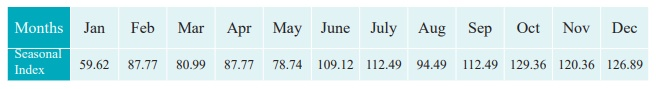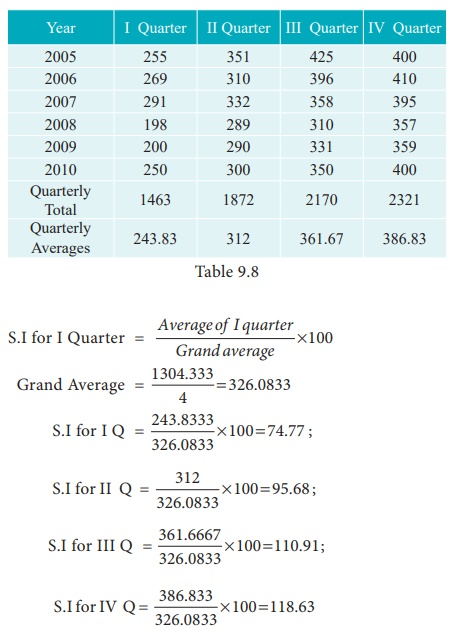Home | | Business Maths 12th Std | Measurements of Trends: Methods of measuring Seasonal Variations By Simple Averages

# Measurements of Trends: Methods of measuring Seasonal Variations By Simple Averages

Seasonal Variations can be measured by the method of simple average. The data should be available in season wise likely weeks, months, quarters.

Methods of measuring Seasonal Variations By Simple Averages :

Seasonal Variations can be measured by the method of simple average. The data should be available in season wise likely weeks, months, quarters.

Method of Simple Averages:

This is the simplest and easiest method for studying Seasonal Variations. The procedure of simple average method is outlined below.

Procedure:

(i) Arrange the data by months, quarters or years according to the data given.

(ii) Find the sum of the each months, quarters or year.

(iii) Find the average of each months, quarters or year.

(iv) Find the average of averages, and it is called Grand Average (G)

(v) Compute Seasonal Index for every season (i.e) months, quarters or year is given by(vi) If the data is given in monthsSimilarly we can calculate SI for all other months.

(vii) If the data is given in quarterExample 9.8

Calculate the seasonal index for the monthly sales of a product using the method of simple averages.Solution:

Computation of seasonal Indices by method of simple averages.Similarly other seasonal index values can be obtained.Example 9.9

Calculate the seasonal index for the quarterly production of a product using the method of simple averages.Solution:

Computation of Seasonal Index by the method of simple averages.Tags : Procedure, Example Solved Problem | Time Series Analysis , 12th Business Maths and Statistics : Chapter 9 : Applied Statistics
Study Material, Lecturing Notes, Assignment, Reference, Wiki description explanation, brief detail
12th Business Maths and Statistics : Chapter 9 : Applied Statistics : Measurements of Trends: Methods of measuring Seasonal Variations By Simple Averages | Procedure, Example Solved Problem | Time Series Analysis